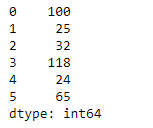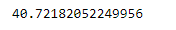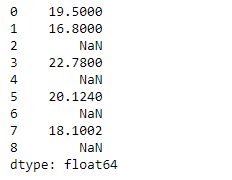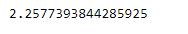Related Articles

# Python | Pandas Series.std()

• Last Updated : 05 Feb, 2019

Pandas series is a One-dimensional ndarray with axis labels. The labels need not be unique but must be a hashable type. The object supports both integer- and label-based indexing and provides a host of methods for performing operations involving the index.

Pandas` Series.std()` function return sample standard deviation over requested axis. The standard deviation is normalized by N-1 by default. This can be changed using the ddof argument.

Syntax: Series.std(axis=None, skipna=None, level=None, ddof=1, numeric_only=None, **kwargs)

Parameter :
axis : {index (0)}
skipna : Exclude NA/null values. If an entire row/column is NA, the result will be NA
level : If the axis is a MultiIndex (hierarchical), count along a particular level, collapsing into a scalar
ddof : Delta Degrees of Freedom. The divisor used in calculations is N – ddof, where N represents the number of elements.
numeric_only : boolean, default None

Returns : std : scalar or Series (if level specified)

Example #1 : Use `Series.std()` function to find the standard deviation of the given Series object.

 `# importing pandas as pd``import` `pandas as pd`` ` `# Creating the Series``sr ``=` `pd.Series([``100``, ``25``, ``32``, ``118``, ``24``, ``65``])`` ` `# Print the series``print``(sr)`

Output :Now we will use `Series.std()` function to find the standard deviation of the given Series object.

 `# find standard-deviation along the``# 0th index``sr.std()`

Output :As we can see in the output, `Series.std()` function has successfully calculated the standard deviation the given Series object.

Example #2 : Use `Series.std()` function to find the standard deviation of the given Series object. We have some missing values in our series object, so skip those missing values.

 `# importing pandas as pd``import` `pandas as pd`` ` `# Creating the Series``sr ``=` `pd.Series([``19.5``, ``16.8``, ``None``, ``22.78``, ``None``, ``20.124``, ``None``, ``18.1002``, ``None``])`` ` `# Print the series``print``(sr)`

Output :Now we will use `Series.std()` function to find the standard deviation of the given Series object.

 `# find standard-deviation along the``# 0th index``sr.std(skipna ``=` `True``)`

Output :As we can see in the output, `Series.std()` function has successfully calculated the standard deviation the given Series object. If we do not skip the missing values then the output will be `NaN`.

Attention geek! Strengthen your foundations with the Python Programming Foundation Course and learn the basics.

To begin with, your interview preparations Enhance your Data Structures concepts with the Python DS Course. And to begin with your Machine Learning Journey, join the Machine Learning – Basic Level Course

My Personal Notes arrow_drop_up